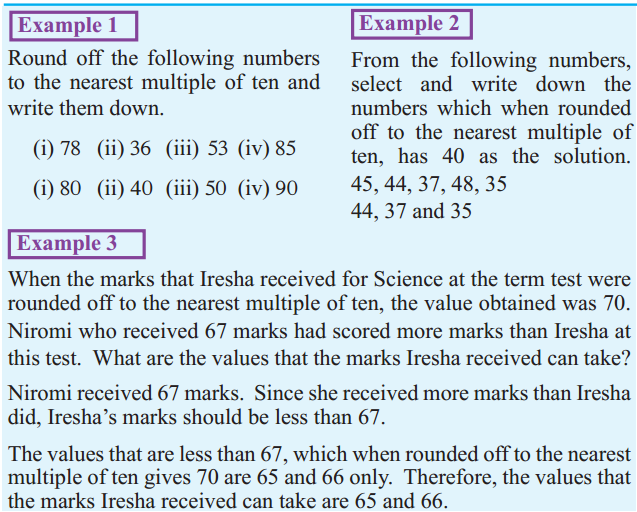# Grade 6 – Unit 06 – Rounding Off

Write this note in your exercise book.

## Rounding Off

When rounding off a number to the nearest multiple of ten, if the digit in the ones place of the number

• is less than 5 (1,2,3,4), then the closest multiple of ten which is less than that number is selected.
• is 5 or greater than 5 (5,6,7,8,9), then the closest multiple of ten which is greater than that number is selected.

Try to understand following examples.### Do the exercise 6.2 in the page number 82 and 83

(Do not write the questions.)

NOTE :
This lesson is targeted for Sri Lankan students.